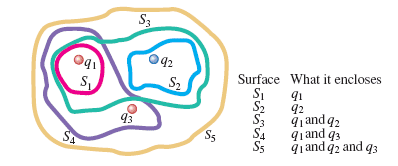# Gauss's Law, net electric flux

• yaro99

## Homework Statement

The three small spheres shown in the figure below carry charges q1 = 4.30 nC, q2 = -7.70 nC, and q3 = 3.40 nC.

Find the net electric flux through each of the following closed surfaces shown in cross section in the figure.## Homework Equations

$\Phi$E=$\frac{Q_{encl}}{\epsilon_0}$

## The Attempt at a Solution

Using the above equation, I got the right answers for S1 through S4, but I'm getting the wrong answer for S5:
$\Phi$E=$\frac{Q_{encl}}{\epsilon_0}$= $\frac{4.30-7.70+3.40}{\epsilon_0}$= $\frac{0}{\epsilon_0}$=0